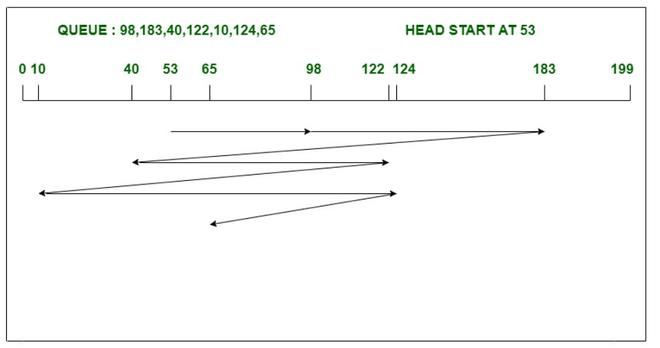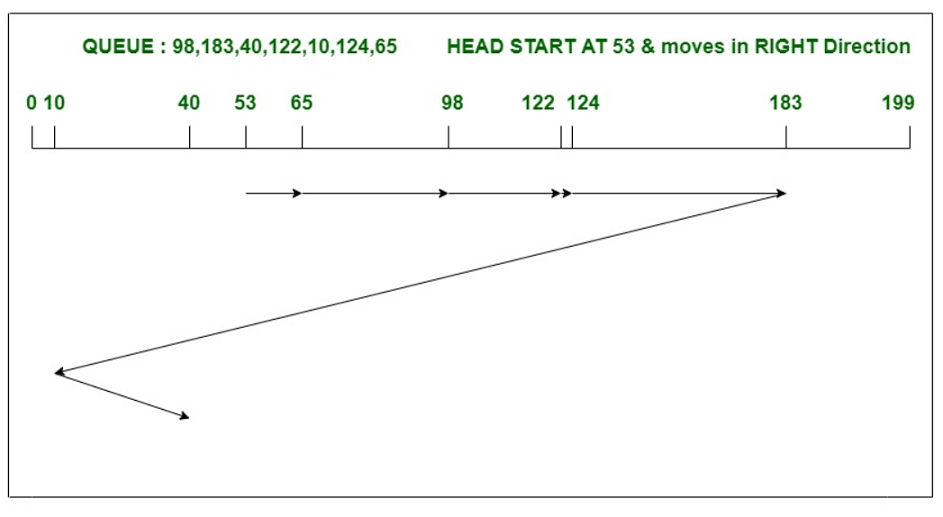# Difference between FCFS and C-LOOK disk scheduling algorithm

• Last Updated : 21 Apr, 2022

### 1. FCFS Disk Scheduling Algorithm

FCFS stands for First Come First Serve, this algorithm entertains the task in the order they arrived in the disk queue. It is the simplest and easy to understand disk scheduling algorithm. In this, the head or pointer moves in the direction in which the task arrives and moves till all request is served. However, the FCFS algorithm has a more fair policy of handling upcoming requests. FCFS algorithm lags in performance in comparison to all the remaining disk scheduling algorithms.

Example:

Consider a disk with 200 tracks (0-199) and the disk queue having I/O requests in the following order as follows: 98, 183, 40, 122, 10, 124, 65. The current head position of the Read\Write head is 53. Calculate the total number of track movements of Read/Write head using FCFS algorithm.```Total head movements
= (98-53)+(183-98)+(183-40)
+(122-40)+(122-10)+(124-10)+(124-65)
= 640```

### 2. C-LOOK Disk Scheduling Algorithm

C-LOOK is the modified version of the LOOK algorithm. In this algorithm, the head starts from the first request in one direction and moves towards the last request at the other end, serving all requests in between. After reaching the last request in one end, the head jumps in another direction and move towards the remaining requests and then satisfies them in the same direction as before. C-LOOK algorithm serves the requests only in one direction. Unlike FCFS, it doesn’t serve requests in order of there arrival.

Example :

Consider a disk with 200 tracks (0-199) and the disk queue having I/O requests in the following order as follows: 98, 183, 40, 122, 10, 124, 65. The current head position of the Read/Write head is 53 and will move in Right direction. Calculate the total number of track movements of Read/Write head using C-LOOK algorithm.```Total head movements
= (65-53)+(98-65)+(122-98)
+(124-122)+(183-124)+(183-10)+(40-10)
= 333```

Difference between FCFS and C-LOOK Disk Scheduling Algorithm :

My Personal Notes arrow_drop_up